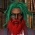Display replies flat, with oldest first Display replies flat, with newest first Display replies in threaded form Display replies in nested formHarald Woracek (Vienna University of Technology) , "High-energy behavior of the Weyl coefficient of a canonical system"by Egor Pifagorov - Saturday, 18 January 2020, 08:55 PM КОЛЛОКВИУМ ФАКУЛЬТЕТА МАТЕМАТИКИ И КОМПЬЮТЕРНЫХ НАУК Факультет математики и компьютерных наук, аудитория 105 (14-я линия В. О., 29)чт. 23 января 17:15Harald Woracek (Vienna University of Technology)High-energy behavior of the Weyl coefficient of a canonical systemWe discuss a topic which occurs in the spectral theory of two-dimensional canonical systems. A canonical system is a differential equation of the form y'(t)=zJH(t)y(t), where the Hamiltonian H is a positive semidefinite 2x2-matrix valued function on the half-line [0,\infty) normalised by tr H(t)=1 a.e.By H.Weyl's nested disks method a function q_H, mapping the upper half-plane C^+ analytically into itself, can be constructed. This function, called the Weyl coefficient, plays an important role: it allows to construct a scalar spectral measure for the differential operator induced by the equation.It is a common meta-principle that the high-energy behaviour of q_H, i.e., its behaviour towards +i\infty, is related to the behaviour of the corresponding Hamiltonian H towards 0. In this talk we instantiate this principle by means of several theorems giving explicit connections q_H towards i\infty and H locally at 0.